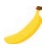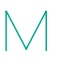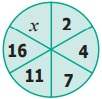Home | | Maths 6th Std | Exercise 2.1

# Exercise 2.1

6th Maths : Term 1 Unit 2 : Introduction To Algebra : Text Book Back Exercises Questions with Answers, Solution

Exercise 2.1

1. Fill in the blanks.

(i) The letters a, b, c, … x, y, z are used to represent _________.  Answer: Variables.

(ii) A quantity that takes ___________ values is called a variable. Answer: Different

(iii) If there are 5 students in a bench, then the number of students in ‘n’ benches is ‘5 × n’. Here ___________ is a variable. Answer: ‘n’

2. Say True or False.

(i)The length of part B in the pencil shown is ‘a − 6’. Answer: [False]

(ii) If the cost of anis ‘x’ and the cost of ais ₹5, then the total cost of fruits is ₹ ' x + 5'Answer: [True]

(iii) If there are 11 players in a team, then there will be '11 + q' players in 'q' teams. Answer: [False]

3. Draw the next two patterns and complete the table.4. Use a variable to write the rule, which gives the number of ice candy sticks required to make the following patterns.

(a) a pattern of letter C as(b) a pattern of letter M as5. The teacher forms groups of five students in a class. How many students will be there in ‘p’ groups?

6. Arivazhagan is 30 years younger to his father. Write Arivazhagan's age in terms of his father's age.

Answer: Arivazhagan’s age = n − 30

7. If ‘u’ is an even number, how would you represent

(i) the next even number? Answer: u + 2

(ii) the previous even number? Answer: u – 2

Objective Type Questions

8. Variable means that it

(a) can take only a few values

(b) has a fixed value

(c) can take different values

(d) can take only 8 values

Answer: (c) can take only a few values

9. ‘6y’ means

(a) 6 + y

(b) 6 – y

(c) 6 × y

(d) 6/y

10. Radha is ‘x’ years of age now. 4 years ago, her age was

(a) x − 4

(b) 4 – x

(c) 4 + x

(d) 4x

11. The number of days in ‘w’ weeks is

(a) 30 + w

(b) 30w

(c) 7 + w

(d) 7w

12. The value of ‘x’ in the circle is

(a) 6

(b) 8

(c) 21

(d) 22Exercise 2.1

1) (i) Variables (ii) Different (iii) n

2) (i) False (ii) True (iii) False

3)4) a) 3n b) 4n

5) 5p

6) Arivazhagan’s age is ‘n-30’

7) (i) u + 2 (ii) u – 2

Objective Type Questions

8) c) can take different values

9) c) 6 × y

10) a) x − 4

11) d) 7w

12) d) 22

Tags : Questions with Answers, Solution | Introduction to Algebra | Term 1 Chapter 2 | 6th Maths , 6th Maths : Term 1 Unit 2 : Introduction To Algebra
Study Material, Lecturing Notes, Assignment, Reference, Wiki description explanation, brief detail
6th Maths : Term 1 Unit 2 : Introduction To Algebra : Exercise 2.1 | Questions with Answers, Solution | Introduction to Algebra | Term 1 Chapter 2 | 6th Maths